# verde.train_test_split¶

verde.train_test_split(coordinates, data, weights=None, **kwargs)[source]

Split a dataset into a training and a testing set for cross-validation.

Similar to sklearn.model_selection.train_test_split but is tuned to work on multi-component spatial data with optional weights.

Extra keyword arguments will be passed to sklearn.model_selection.ShuffleSplit, except for n_splits which is always 1.

Parameters: coordinates : tuple of arrays Arrays with the coordinates of each data point. Should be in the following order: (easting, northing, vertical, …). data : array or tuple of arrays the data values of each data point. If the data has more than one component, data must be a tuple of arrays (one for each component). weights : none or array or tuple of arrays if not none, then the weights assigned to each data point. If more than one data component is provided, you must provide a weights array for each data component (if not none). train, test : tuples Each is a tuple = (coordinates, data, weights) generated by separating the input values randomly.

Examples

>>> import numpy as np
>>> # Split 2-component data with weights
>>> data = (np.array([1, 3, 5, 7]), np.array([0, 2, 4, 6]))
>>> coordinates = (np.arange(4), np.arange(-4, 0))
>>> weights = (np.array([1, 1, 2, 1]), np.array([1, 2, 1, 1]))
>>> train, test = train_test_split(coordinates, data, weights,
...                                random_state=0)
>>> print("Coordinates:", train, test, sep='\n  ')
Coordinates:
(array([3, 1, 0]), array([-1, -3, -4]))
(array(), array([-2]))
>>> print("Data:", train, test, sep='\n  ')
Data:
(array([7, 3, 1]), array([6, 2, 0]))
(array(), array())
>>> print("Weights:", train, test, sep='\n  ')
Weights:
(array([1, 1, 1]), array([1, 2, 1]))
(array(), array())
>>> # Split single component data without weights
>>> train, test = train_test_split(coordinates, data, None,
...                                random_state=0)
>>> print("Coordinates:", train, test, sep='\n  ')
Coordinates:
(array([3, 1, 0]), array([-1, -3, -4]))
(array(), array([-2]))
>>> print("Data:", train, test, sep='\n  ')
Data:
(array([7, 3, 1]),)
(array(),)
>>> print("Weights:", train, test, sep='\n  ')
Weights:
(None,)
(None,)


## Examples using verde.train_test_split¶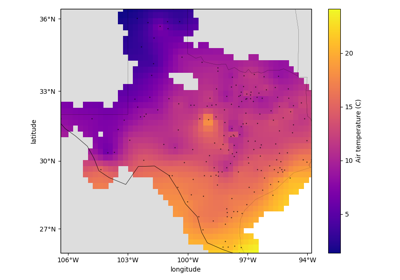Gridding with splines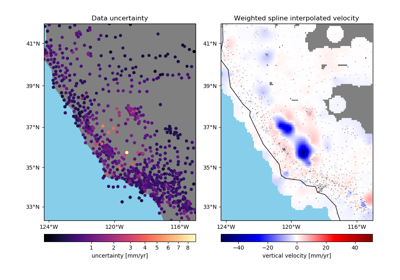Gridding with splines and weights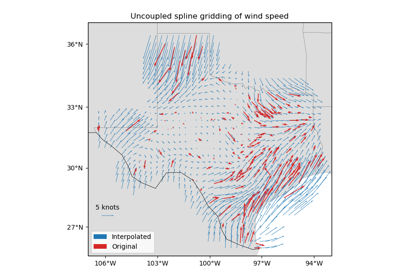Gridding 2D vectors (uncoupled)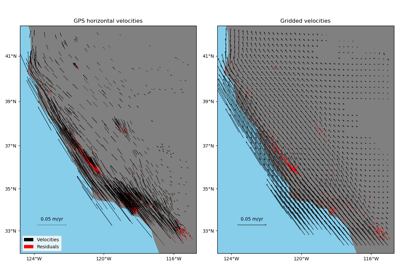Gridding 2D vectors (coupled)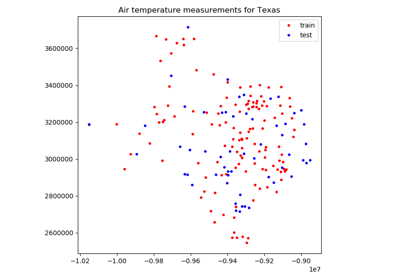Model Selection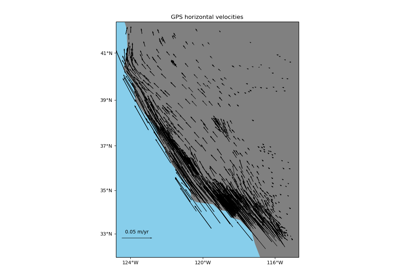Vector Data